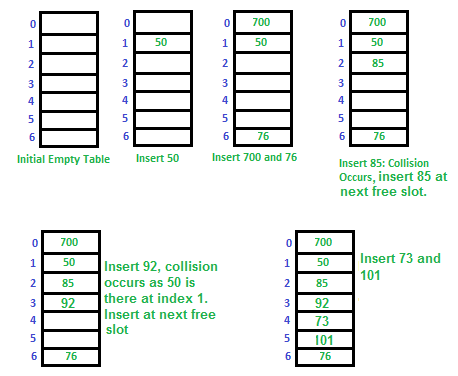# Hashing | Set 3 (Open Addressing)

• Difficulty Level : Easy
• Last Updated : 14 Jul, 2022

We strongly recommend referring below post as a prerequisite of this.
Hashing | Set 1 (Introduction)
Hashing | Set 2 (Separate Chaining)

Like separate chaining, open addressing is a method for handling collisions. In Open Addressing, all elements are stored in the hash table itself. So at any point, the size of the table must be greater than or equal to the total number of keys (Note that we can increase table size by copying old data if needed). This approach is also known as closed hashing. This entire procedure is based upon probing. We will understand types of probing ahead.• Insert(k): Keep probing until an empty slot is found. Once an empty slot is found, insert k.
• Search(k): Keep probing until slot’s key doesn’t become equal to k or an empty slot is reached.
• Delete(k): Delete operation is interesting. If we simply delete a key, then the search may fail. So slots of deleted keys are marked specially as “deleted”.
The insert can insert an item in a deleted slot, but the search doesn’t stop at a deleted slot.

Open Addressing is done in the following ways:

a) Linear Probing:

In linear probing, the hash table is searched sequentially that starts from the original location of the hash. If in case the location that we get is already occupied, then we check for the next location.
The function used for rehashing is as follows: rehash(key) = (n+1)%table-size. For example, the typical gap between two probes is 1 as seen in the example below.
Let hash(x) be the slot index computed using a hash function and S be the table size

```If slot hash(x) % S is full, then we try (hash(x) + 1) % S
If (hash(x) + 1) % S is also full, then we try (hash(x) + 2) % S
If (hash(x) + 2) % S is also full, then we try (hash(x) + 3) % S
..................................................
..................................................```

Let us consider a simple hash function as “key mod 7” and a sequence of keys as 50, 700, 76, 85, 92, 73, 101.Challenges in Linear Probing :

1. Primary Clustering: One of the problems with linear probing is Primary clustering, many consecutive elements form groups and it starts taking time to find a free slot or to search for an element.
2. Secondary Clustering: Secondary clustering is less severe, two records only have the same collision chain (Probe Sequence) if their initial position is the same.

b) Quadratic Probing If you observe carefully, then you will understand that the interval between probes will increase proportionally to the hash value. Quadratic probing is a method with the help of which we can solve the problem of clustering that was discussed above.  This method is also known as mid-square method. In this method we look for i2‘th slot in i’th iteration.  We always start from the original hash location. If only the location is occupied then we check the other slots.

```let hash(x) be the slot index computed using hash function.
If slot hash(x) % S is full, then we try (hash(x) + 1*1) % S
If (hash(x) + 1*1) % S is also full, then we try (hash(x) + 2*2) % S
If (hash(x) + 2*2) % S is also full, then we try (hash(x) + 3*3) % S
..................................................
..................................................```

c) Double Hashing The intervals that lie between probes is computed by another hash function. Double hashing is a technique that reduces clustering in an optimized way. In this technique, the increments for the probing sequence are computed by using another hash function. We use another hash function hash2(x) and look for i*hash2(x) slot in i’th rotation.

```let hash(x) be the slot index computed using hash function.
If slot hash(x) % S is full, then we try (hash(x) + 1*hash2(x)) % S
If (hash(x) + 1*hash2(x)) % S is also full, then we try (hash(x) + 2*hash2(x)) % S
If (hash(x) + 2*hash2(x)) % S is also full, then we try (hash(x) + 3*hash2(x)) % S
..................................................
..................................................```

See this for step by step diagrams.

Comparison of above three:

• Linear probing has the best cache performance but suffers from clustering. One more advantage of Linear probing is easy to compute.
• Quadratic probing lies between the two in terms of cache performance and clustering.
• Double hashing has poor cache performance but no clustering. Double hashing requires more computation time as two hash functions need to be computed.

Note: Cache performance of chaining is not good because when we traverse a Linked List, we are basically jumping from one node to another, all across the computer’s memory. For this reason, the CPU cannot cache the nodes which aren’t visited yet, this doesn’t help us. But with Open Addressing, data isn’t spread, so if the CPU detects that a segment of memory is constantly being accessed, it gets cached for quick access.

Like Chaining, the performance of hashing can be evaluated under the assumption that each key is equally likely to be hashed to any slot of the table (simple uniform hashing)

```m = Number of slots in the hash table
n = Number of keys to be inserted in the hash table

Load factor α = n/m  ( < 1 )

Expected time to search/insert/delete < 1/(1 - α)

So Search, Insert and Delete take (1/(1 - α)) time```

My Personal Notes arrow_drop_up